# R S Aggarwal Solutions for Class 10 Maths Chapter 15 Perimeter and Area of Plane Figures Exercise 15A

R S Aggarwal Solutions for Class 10 Maths Chapter 15 Perimeter and Area of Plane Figures exercise 15A is provided here. This study material helps students to master problems related to perimeter and area of plane figures. We have provided diagrams also wherever necessary to promote visual learning and better understanding. Download for free R S Aggarwal Solutions of Class 10 Chapter 15 pdf and solve all the questions to get good marks in the exams.

## Download PDF of R S Aggarwal Solutions for Class 10 Maths Chapter 15 Perimeter and Area of Plane Figures Exercise 15A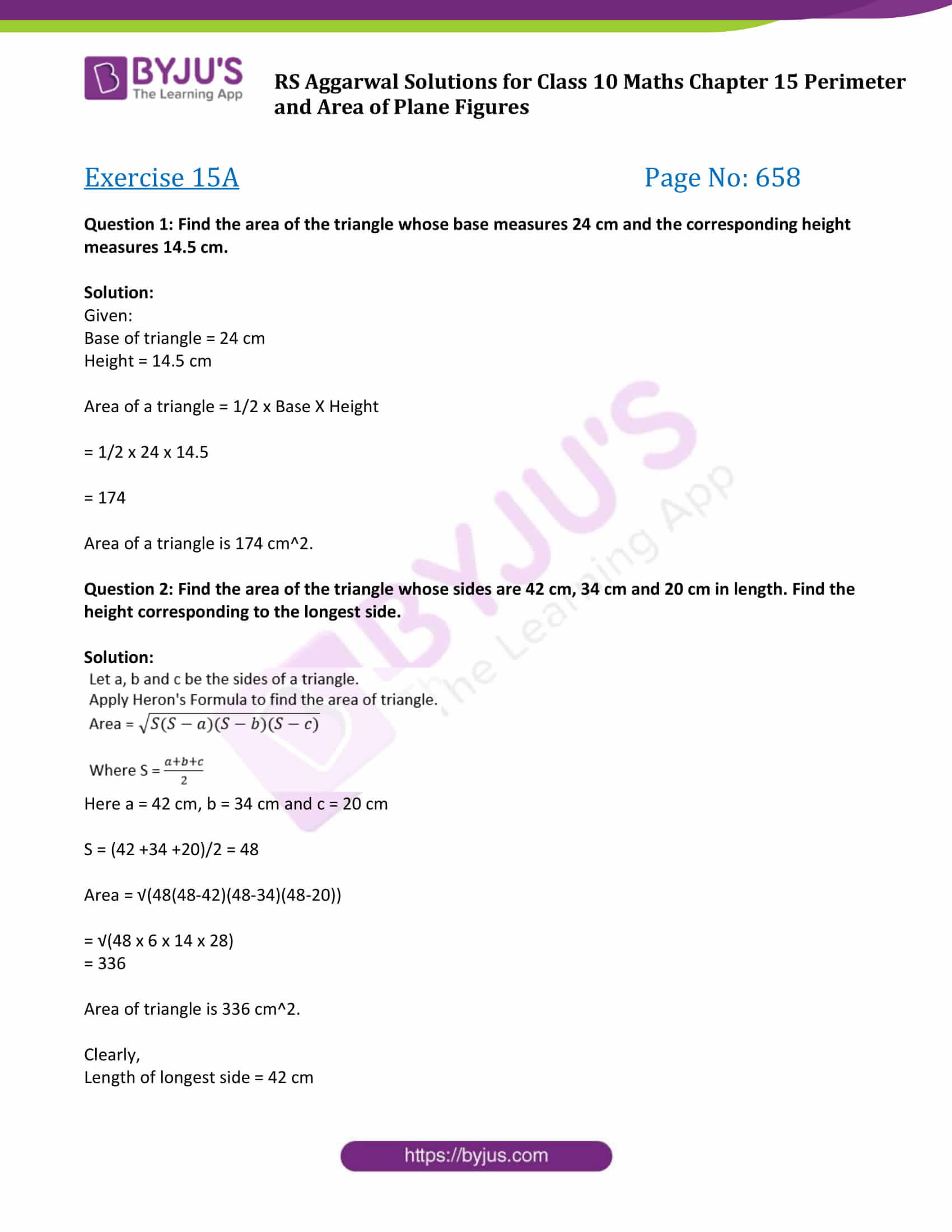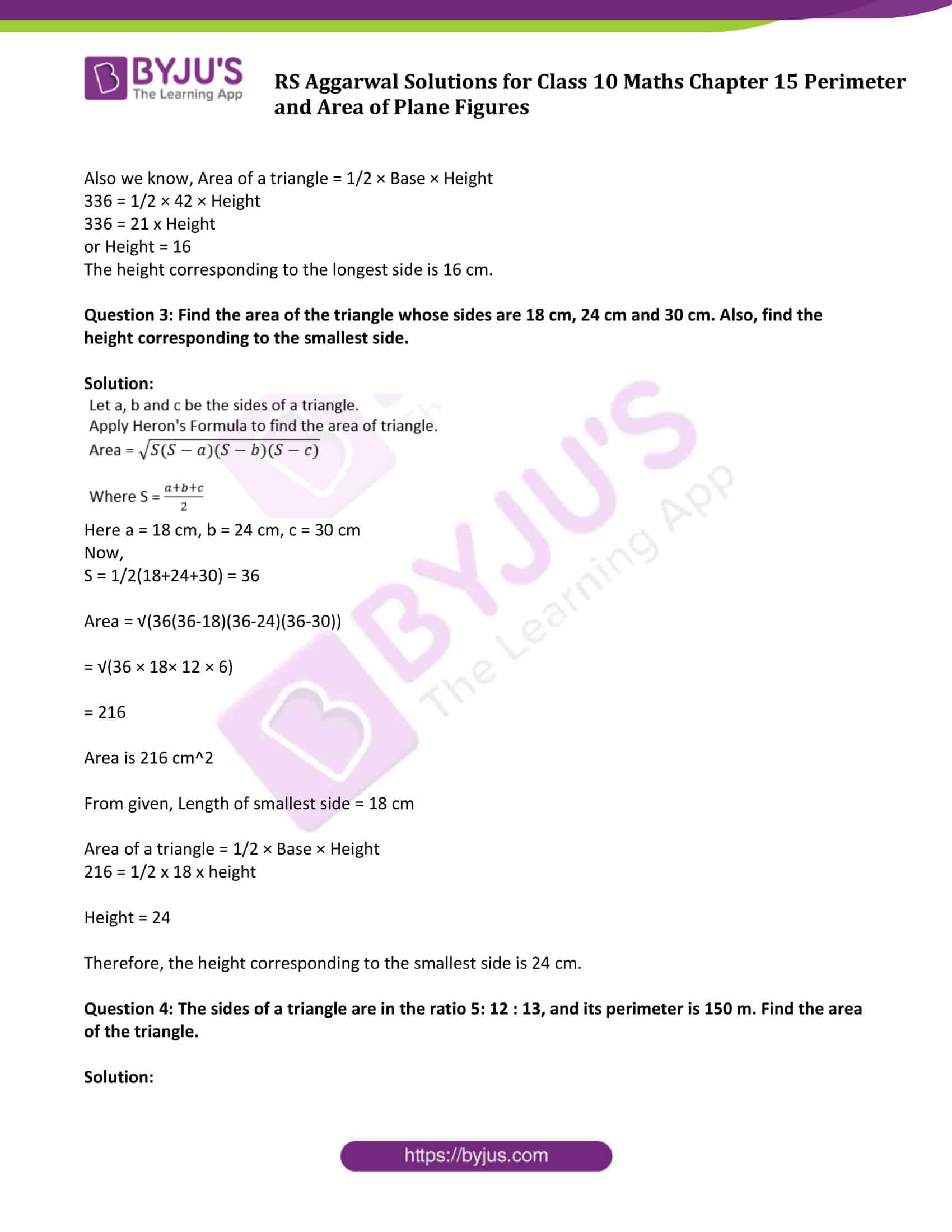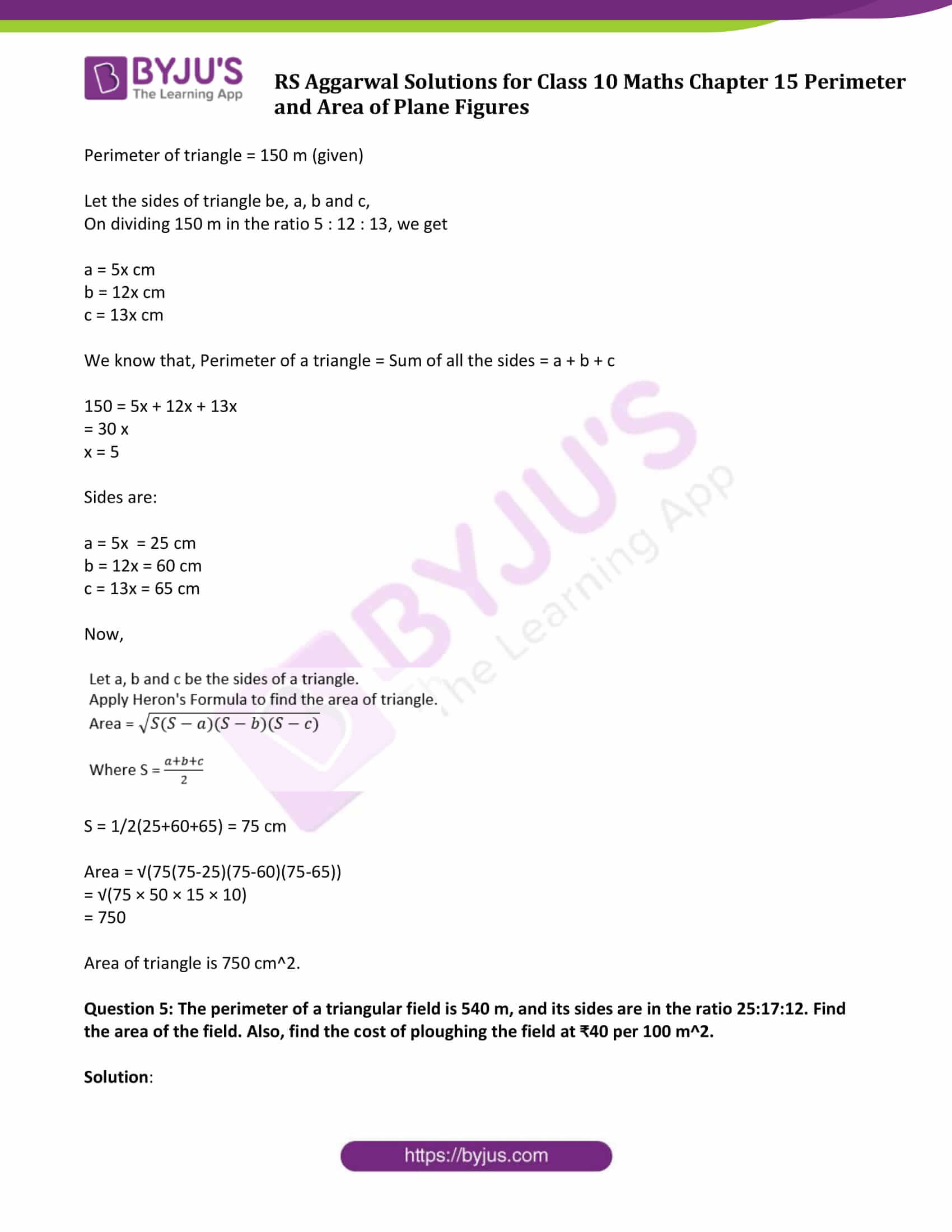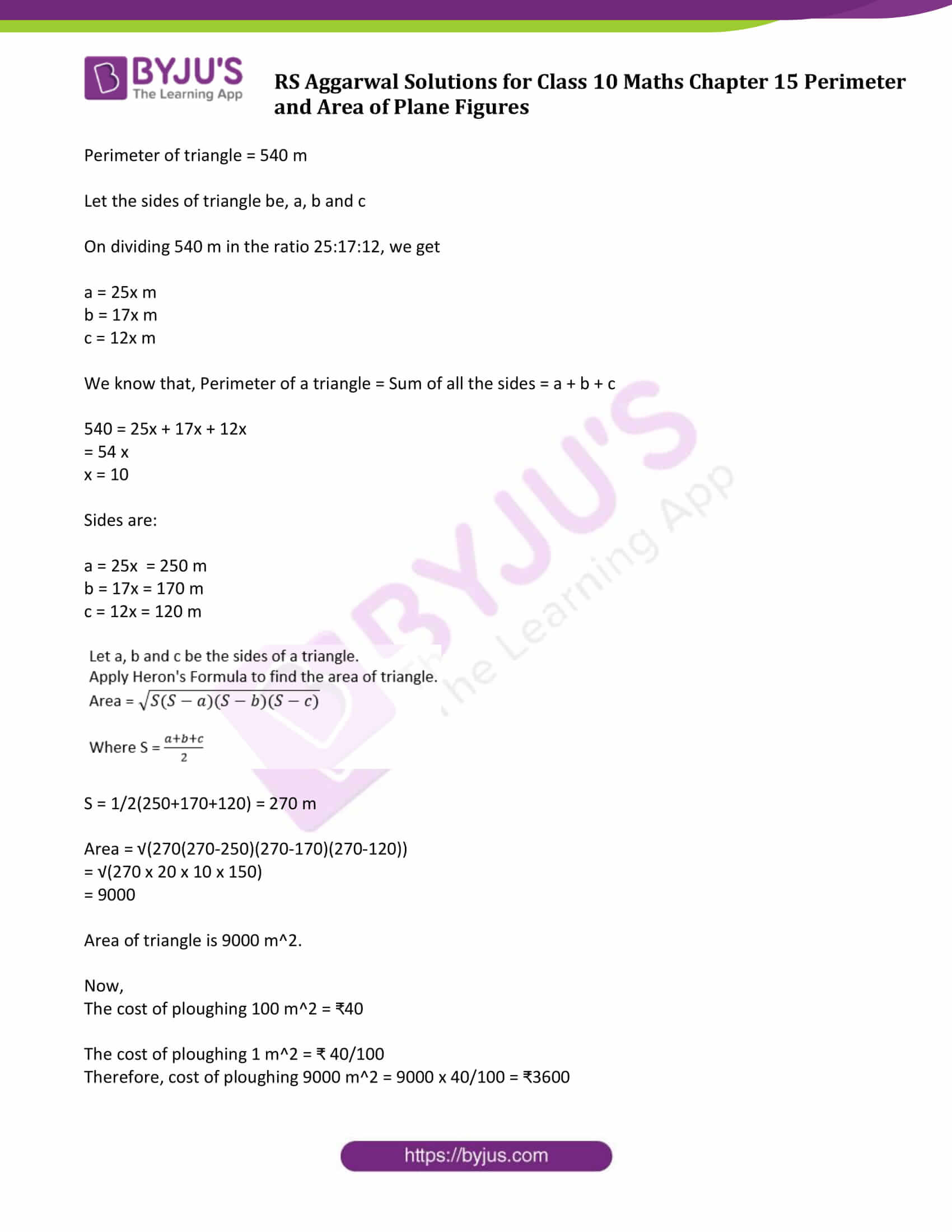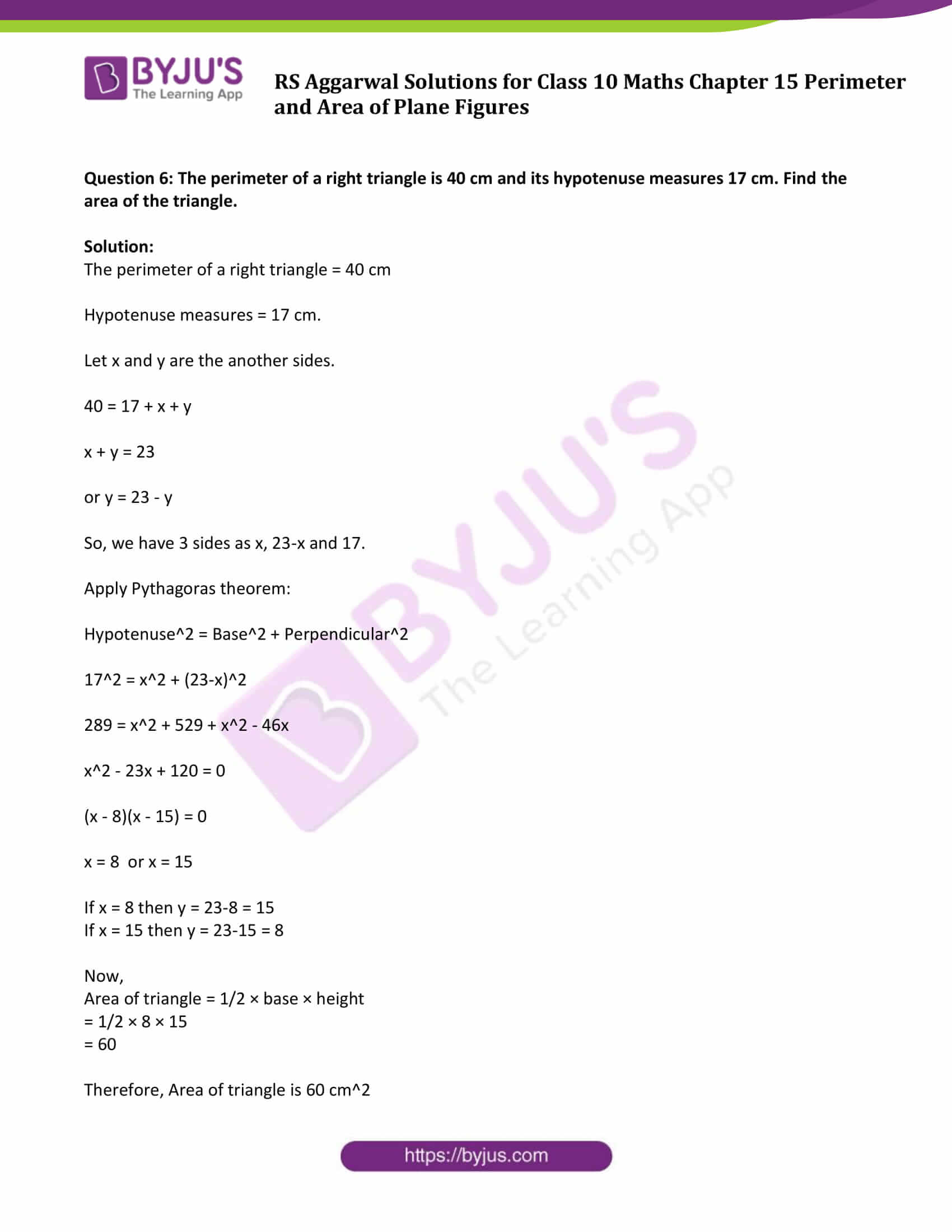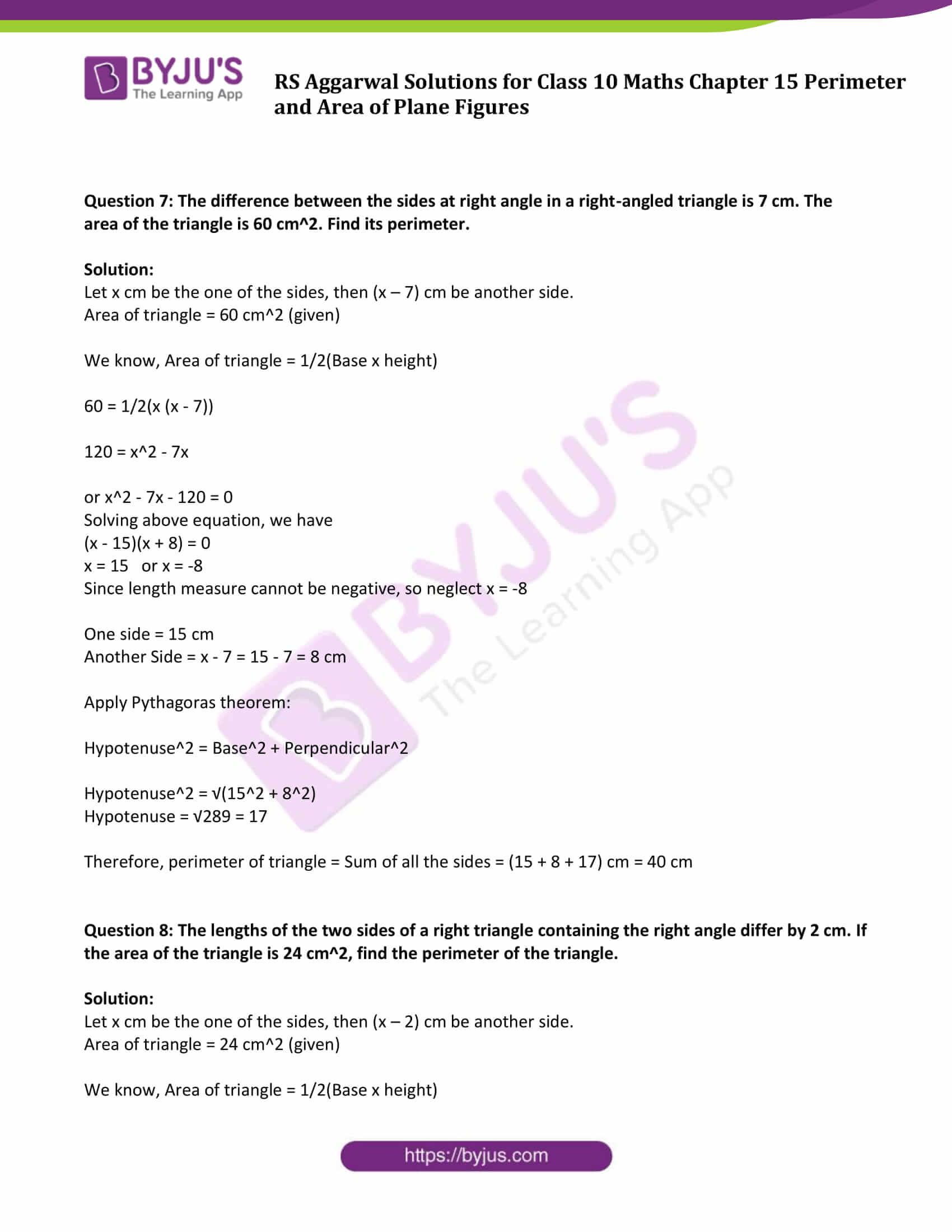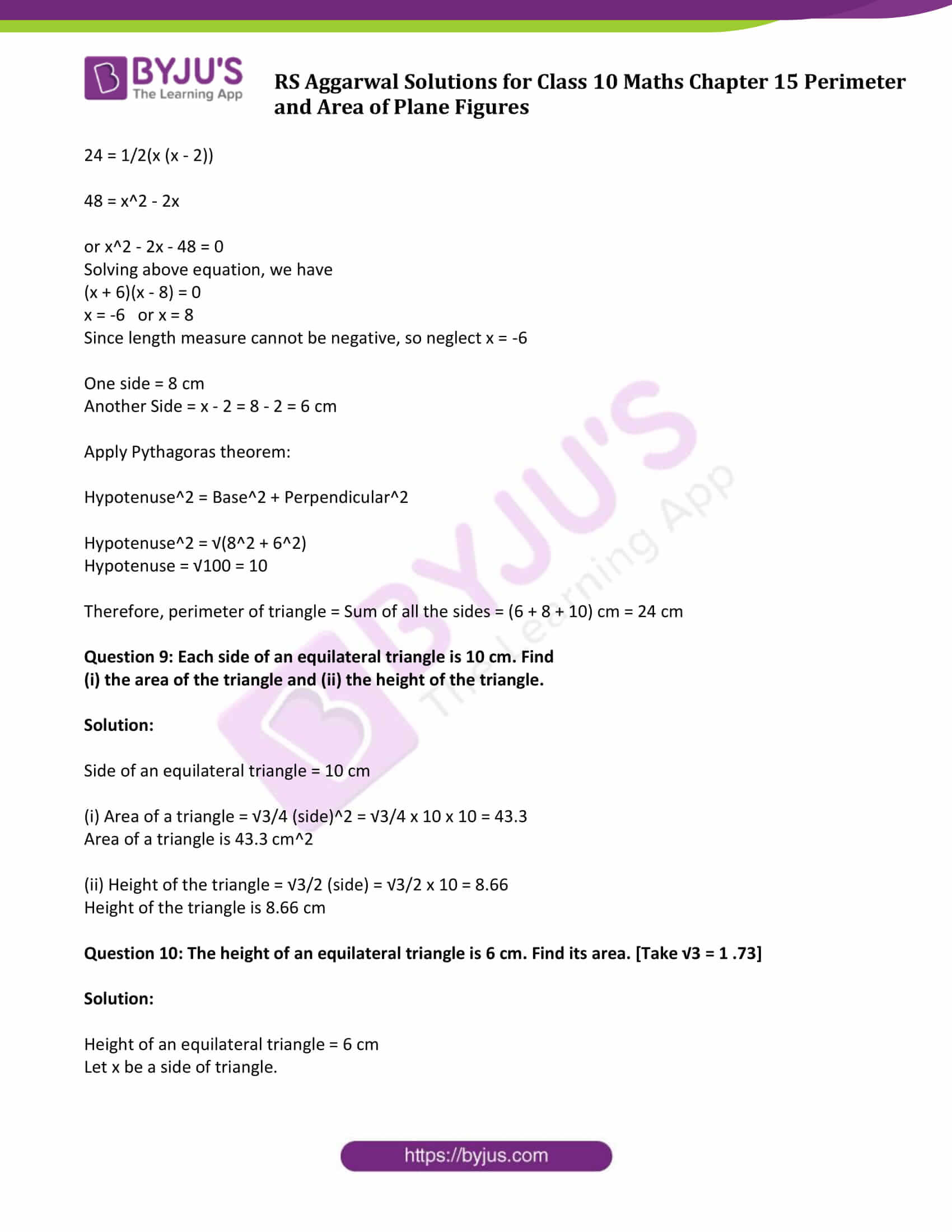### Access other exercise solutions of Class 10 Maths Chapter 15 Perimeter and Area of Plane Figures

Exercise 15 B Solutions

### Access Answers to Maths R S Aggarwal Class 10 Chapter 15 – Perimeter and Area of Plane Figures Exercise 15A

Question 1: Find the area of the triangle whose base measures 24 cm and the corresponding height measures 14.5 cm.
Solution:
Given:
Base of triangle = 24 cm
Height = 14.5 cm
Area of a triangle = 1/2 x Base X Height
= 1/2 x 24 x 14.5
= 174
Area of a triangle is 174 cm^2.
Question 2: Find the area of the triangle whose sides are 42 cm, 34 cm and 20 cm in length. Find the height corresponding to the longest side.
Solution: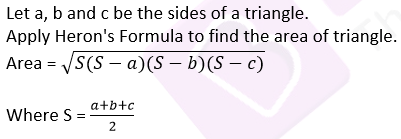Here a = 42 cm, b = 34 cm and c = 20 cm
S = (42 +34 +20)/2 = 48
Area = √(48(48-42)(48-34)(48-20))
= √(48 x 6 x 14 x 28)
= 336
Area of triangle is 336 cm^2.
Clearly,
Length of longest side = 42 cm
Also we know, Area of a triangle = 1/2 × Base × Height
336 = 1/2 × 42 × Height
336 = 21 x Height
or Height = 16
The height corresponding to the longest side is 16 cm.
Question 3: Find the area of the triangle whose sides are 18 cm, 24 cm and 30 cm. Also, find the height corresponding to the smallest side.
Solution:Here a = 18 cm, b = 24 cm, c = 30 cm
Now,
S = 1/2(18+24+30) = 36
Area = √(36(36-18)(36-24)(36-30))
= √(36 × 18× 12 × 6)
= 216
Area is 216 cm^2
From given, Length of smallest side = 18 cm
Area of a triangle = 1/2 × Base × Height
216 = 1/2 x 18 x height
Height = 24
Therefore, the height corresponding to the smallest side is 24 cm.
Question 4: The sides of a triangle are in the ratio 5: 12 : 13, and its perimeter is 150 m. Find the area of the triangle.
Solution:
Perimeter of triangle = 150 m (given)
Let the sides of triangle be, a, b and c,
On dividing 150 m in the ratio 5 : 12 : 13, we get
a = 5x cm
b = 12x cm
c = 13x cm
We know that, Perimeter of a triangle = Sum of all the sides = a + b + c
150 = 5x + 12x + 13x
= 30 x
x = 5
Sides are:
a = 5x = 25 cm
b = 12x = 60 cm
c = 13x = 65 cm
Now,S = 1/2(25+60+65) = 75 cm
Area = √(75(75-25)(75-60)(75-65))
= √(75 × 50 × 15 × 10)
= 750
Area of triangle is 750 cm^2.
Question 5: The perimeter of a triangular field is 540 m, and its sides are in the ratio 25:17:12. Find the area of the field. Also, find the cost of ploughing the field at ₹40 per 100 m^2.
Solution:
Perimeter of triangle = 540 m
Let the sides of triangle be, a, b and c
On dividing 540 m in the ratio 25:17:12, we get
a = 25x m
b = 17x m
c = 12x m
We know that, Perimeter of a triangle = Sum of all the sides = a + b + c
540 = 25x + 17x + 12x
= 54 x
x = 10
Sides are:
a = 25x = 250 m
b = 17x = 170 m
c = 12x = 120 mS = 1/2(250+170+120) = 270 m
Area = √(270(270-250)(270-170)(270-120))
= √(270 x 20 x 10 x 150)
= 9000
Area of triangle is 9000 m^2.
Now,
The cost of ploughing 100 m^2 = ₹40
The cost of ploughing 1 m^2 = ₹ 40/100
Therefore, cost of ploughing 9000 m^2 = 9000 x 40/100 = ₹3600
Question 6: The perimeter of a right triangle is 40 cm and its hypotenuse measures 17 cm. Find the area of the triangle.
Solution:
The perimeter of a right triangle = 40 cm
Hypotenuse measures = 17 cm.
Let x and y are the another sides.
40 = 17 + x + y
x + y = 23
or y = 23 – y
So, we have 3 sides as x, 23-x and 17.
Apply Pythagoras theorem:
Hypotenuse^2 = Base^2 + Perpendicular^2
17^2 = x^2 + (23-x)^2
289 = x^2 + 529 + x^2 – 46x
x^2 – 23x + 120 = 0
(x – 8)(x – 15) = 0
x = 8 or x = 15
If x = 8 then y = 23-8 = 15
If x = 15 then y = 23-15 = 8
Now,
Area of triangle = 1/2 × base × height
= 1/2 × 8 × 15
= 60
Therefore, Area of triangle is 60 cm^2
Question 7: The difference between the sides at right angle in a right-angled triangle is 7 cm. The area of the triangle is 60 cm^2. Find its perimeter.
Solution:
Let x cm be the one of the sides, then (x – 7) cm be another side.
Area of triangle = 60 cm^2 (given)
We know, Area of triangle = 1/2(Base x height)
60 = 1/2(x (x – 7))
120 = x^2 – 7x
or x^2 – 7x – 120 = 0
Solving above equation, we have
(x – 15)(x + 8) = 0
x = 15 or x = -8
Since length measure cannot be negative, so neglect x = -8
One side = 15 cm
Another Side = x – 7 = 15 – 7 = 8 cm
Apply Pythagoras theorem:
Hypotenuse^2 = Base^2 + Perpendicular^2
Hypotenuse^2 = √(15^2 + 8^2)
Hypotenuse = √289 = 17
Therefore, perimeter of triangle = Sum of all the sides = (15 + 8 + 17) cm = 40 cm
Question 8: The lengths of the two sides of a right triangle containing the right angle differ by 2 cm. If the area of the triangle is 24 cm^2, find the perimeter of the triangle.
Solution:
Let x cm be the one of the sides, then (x – 2) cm be another side.
Area of triangle = 24 cm^2 (given)
We know, Area of triangle = 1/2(Base x height)
24 = 1/2(x (x – 2))
48 = x^2 – 2x
or x^2 – 2x – 48 = 0
Solving above equation, we have
(x + 6)(x – 8) = 0
x = -6 or x = 8
Since length measure cannot be negative, so neglect x = -6
One side = 8 cm
Another Side = x – 2 = 8 – 2 = 6 cm
Apply Pythagoras theorem:
Hypotenuse^2 = Base^2 + Perpendicular^2
Hypotenuse^2 = √(8^2 + 6^2)
Hypotenuse = √100 = 10
Therefore, perimeter of triangle = Sum of all the sides = (6 + 8 + 10) cm = 24 cm
Question 9: Each side of an equilateral triangle is 10 cm. Find
(i) the area of the triangle and (ii) the height of the triangle.
Solution:
Side of an equilateral triangle = 10 cm
(i) Area of a triangle = √3/4 (side)^2 = √3/4 x 10 x 10 = 43.3
Area of a triangle is 43.3 cm^2
(ii) Height of the triangle = √3/2 (side) = √3/2 x 10 = 8.66
Height of the triangle is 8.66 cm
Question 10: The height of an equilateral triangle is 6 cm. Find its area. [Take √3 = 1 .73]
Solution:
Height of an equilateral triangle = 6 cm
Let x be a side of triangle.
Height = √3/2 (side) = √3/2 x
6 = √3/2 x
x = 12/√3
x = 12√3/3 = 6.92 cm
Now,
Area of a triangle = √3/4 (side)^2 = √3/4 x 6.92 x 6.92 = 20.76 cm^2
Question 11: If the area of an equilateral triangle is 36√3 cm^2, find its perimeter.
Solution:
Let each side of an equilateral triangle be “a” cm
Area of an equilateral triangle = 36√3 cm^2 (Given)
Area of an equilateral triangle = √3/4 (side)^2
36√3 = √3/4 x a^2
a^2 = 144
or a = 12 cm
Perimeter = 3 (side) = 3 x 12 = 36 cm.
Question 12: If the area of an equilateral triangle is 81√3 cm^2, find its height.
Solution:
Let each side of an equilateral triangle be “a” cm
Area of an equilateral triangle = 81√3 cm^2 (Given)
Area of an equilateral triangle = √3/4 (side)^2
81√3 = √3/4 x a^2
a^2 = 324
or a = 18 cm
Now,
Height of an equilateral triangle = √3/2 (side) = √3/4 (18) = 9√3 cm.
Perimeter = 3 (side) = 3 x 12 = 36 cm.
Question 13: The base of a right-angled triangle measures 48 cm and its hypotenuse measures 50 cm. Find the area of the triangle.
Solution:
We are given with a right-angled triangle whose measures are
Base = 48 cm
Hypotenuse = 50 cm
Using Pythagoras Theorem:
Hypotenuse^2 = Base^2 + Perpendicular^2
50^2 = 48^2 + Perpendicular^2
or Perpendicular^2 = 2500 – 2304
or Perpendicular = 14 cm
Now,
Area of a triangle = 1/2 × Base × Height
= 1/2 × 48 cm × 14 cm
= 336 cm^2
Question 14: The hypotenuse of a right-angled triangle is 65 cm and its base is 60 cm. Find the length of perpendicular and the area of the triangle.
Solution:
We are given with a right-angled triangle whose measures are
Base = 6 m
Hypotenuse = 6.5 m
Using Pythagoras Theorem:
Hypotenuse^2 = Base^2 + Perpendicular^2
(6.5)^2 = (6)^2 + Perpendicular^2
or Perpendicular^2 = 42.25 – 36
or Perpendicular = 2.5 m
Now,
Area of a triangle = 1/2 × Base × Height
= 1/2 × 6 m × 2.5 m
= 7.5 cm^2
Question 15: Find the area of a right-angled triangle, the radius of whose circumcircle measure 8 cm and the altitude drawn to the hypotenuse measures 6 cm.
Solution:
Radius of circle = 8 cm and
Altitude = 6 cm
Since, in a right-angled triangle the hypotenuse is the diameter of circumcircle. (given)
= 2 × 8
= 16
=> Hypotenuse = 16 cm
Area of a triangle = 1/2 × Base × Height
(Consider hypotenuse as base and the altitude to the hypotenuse as height)
= 1/2 × 16 x 6
= 48
=> Area of a triangle is 48 cm^2
Question 16: Find the length of the hypotenuse of an isosceles right-angled triangle whose area is 200 cm^2. Also, find its perimeter. [Given: √2 = 1.41]
Solution:
Let two sides of an isosceles right-angled triangle are of measure “a”.
Area of triangle = 200 cm^2. (given)
200 = 1/2(a^2)
=> a = 20 cm
Now,
Hypotenuse = √(a^2+a^2) = √(2a^2) = √2 a
= 20 √2
= 20 x 1.414
= 28.28
=> Hypotenuse is 28.28 cm
Perimeter of triangle = Sum of all the sides = 2a + Hypotenuse
= 40 + 28.28
= 68.28
=> Perimeter of triangle is 68.28 cm.
Question 17: The base of an isosceles triangle measures 80 cm and its area is 360 cm2. Find the perimeter of the triangle.
Solution:
Let two sides of an isosceles triangle are of measure “a”.
Base = b = 80 cm
Area of triangle = 360 cm^2. (given)
We know, Area of isosceles triangle = 1/4 × b√(4a^2 – b^2)
360 = 1/4 x 80 x √(4a^2 – (80)^2)
360 = 20 x √(4a^2 – 6400)
360 = 20 x 2√(a^2 – 1600)
9 = √(a^2 – 1600)
Squaring both sides
81 = a^2 – 1600
a = 41
=> a = 41 cm
Perimeter of triangle = Sum of all the sides = 41 + 41 + 80 = 162
Therefore, perimeter of triangle is 162 cm.
Question 18: Each of the equal sides of an isosceles triangle measures 2 cm more than its height, and the base of the triangle measures 12 cm. Find the area of the triangle.
Solution:
Let h be the height, then
Each of the equal sides of an isosceles triangle = (h + 2) cm and
Base = 12 cm
Area of a triangle = 1/2 × Base × Height
= 1/2 x 12 x h ….(1)
Area of isosceles triangle = 1/4 x b x √(4a^2 – b^2)
= 1/2 x 12 x √(4(h+2)^2 – 144) …(2)
From (1) and (2), we get
1/2 x 12 x h = 1/2 x 12 x √(4(h+2)^2 – 144)
6h = 3√(4h2 + 16h + 16-144)
2h = √(4h^2 + 16h-128)
4h^2 = 4h^2 + 16h – 128
16h = 128
or h = 8
Height is 8 cm.
Now,
Area of a triangle = 1/2 × Base × Height
= 1/2 × 12 cm × 8 cm
= 1/2 × 96 cm^2
= 48 cm^2.
Question 19: Find the area and perimeter of an isosceles right triangle, each of whose equal sides measures 10 cm. [Given: √2 = 1 .41]
Solution:
Equal sides of measure = 10 cm
Here, base and perpendicular are equal sides.
Area of a triangle = 1/2 × Base × Height
= 1/2 x 10 x 10
= 50
From Pythagorean Theorem:
Hypotenuse^2 = Base^2 + Perpendicular^2
= 10^2 + 10^2
= 200
or Hypotenuse = 10 v2 cm = 10 x 1 .41 cm = 14.1 cm
Perimeter = Sum of all the sides = 10 + 10 + 14.1 = 34.1
Therefore, Area of required triangle is 50 cm^2 and perimeter = 34.1 cm
Question 20: In the given figure, ∆ABC is an equilateral triangle the length of whose side is equal to 10 cm, and ∆DBC is right-angled at D and BD = 8 cm. Find the area of the shaded region. [Take √3 = 1.732.]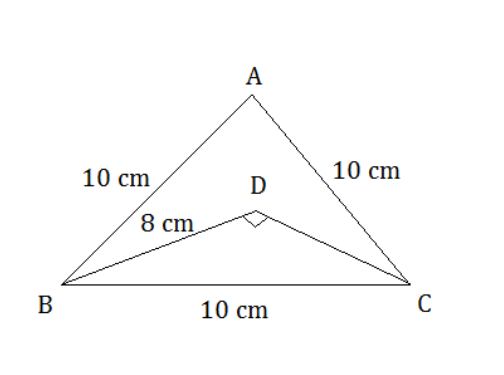Solution:
∆ABC is an equilateral triangle the length of whose side is equal to 10 cm, and ∆DBC is right-angled at D and BD = 8 cm.
From figure:
Area of shaded region = Area of ABC – Area of DBC …..(1)
Area of △ABC:
Area = v3/4 (side)^2 = v3/4 (10)^2 = 43.30
So area of △ABC is 43.30 cm^2
Area of right △DBC:
Area = 1/2 x base x height …(2)
From Pythagorean Theorem:
Hypotenuse^2 = Base^2 + Height^2
BC^2 = DB^2 + Height^2
100 – 64 = Height^2
36 = Height^2
or Height = 6
equation (2) =>
Area = 1/2 x 8 x 6 = 24
So area of △DBC is 24 cm^2
Equation (1) implies
Area of shaded region = 43.30 – 24 = 19.30
Therefore, Area of shaded region = 19.3 cm^2

## R S Aggarwal Solutions for Class 10 Maths Chapter 15 Perimeter and Area of Plane Figures Exercise 15A

Class 10 Maths Chapter 15 Exercise 15A is based on area and perimeter of plane figures.
Some of the important formulas are listed below:
Perimeter of rectangle = 2(length + breadth)
Area of rectangle = length x breadth
Perimeter of square = 4 Side
Area of square = (Side)2
Perimeter of triangle = Sum of all the sides
Area of triangle (when sides (a,b,c) are given)
A = √(s(s – a) (s – b) (s – c)); where S = 1/2 (a + b + c)
Area of triangle = 1/2 × base × height)
Area of an equilateral triangle = √3/4 (side)2